library(knitr)
opts_chunk$set(fig.height=3, fig.path='Figs/', echo=TRUE, warning=FALSE, message=FALSE) #In addition to the libraries listed below,, the Genexpi-stan package requires: # rstan, deSolve, splines, truncnorm, parallel, loo devtools::load_all() library(cowplot) library(tidyverse) library(here) library(MVN) options(mc.cores = parallel::detectCores()) rstan_options(auto_write = TRUE) # Abstract In determining regulatory interactions within cells, models based on ordinary differential equations (ODE) are a popular choice. Here, we present a reimplementation of one such model in Stan. The model features a spline fit where the resulting spline is a parameter of the ODE. For practical reasons, the model avoids the Stan’s ODE solver in favor of a custom solution. We also discuss why the model as traditionally formulated is not well identified and introduce reparametrizations that improve identifiability and make it easier to specify reasonable default priors. This notebook shows first usable version of the model, but there are still several outstanding issues which we highlight. We nevertheless hope that the modelling approach we took is of interest to the Stan community and can provide inspiration for other models. A runnable version of this Rmd file and all supporting code can be found at https://github.com/cas-bioinf/genexpi-stan/tree/stancon2018 # Biological Background In a very simplified form the central dogma of molecular biology may be paraphrased as follows: The DNA contains all of the instructions needed to run a cell and can be divided into coding and non-coding regions. The coding regions of DNA can be transcribed to form messenger RNA (mRNA) molecules containing a mirror-copy of the coding region. The messenger RNA is then translated to create protein. Proteins perform most of the actual functions of the cells, including control of transcription and translation. This process is regulated at all stages and involves many feedback loops: most importantly, the rate of transcription of a gene is controlled by the abundance of regulatory proteins binding to specific sequences of the DNA near the coding region. The rate of translation and degradation of mRNA can also be regulated separately by other proteins and the rate of degradation of proteins themselves is also affected by other proteins. There are many exceptions where the above simplification does not hold, but the vast majority of cellular life can be described in these terms. Understanding what are the regulations taking place is thus an important step in understanding how cells work. However, our ability to observe what happens in the cell is very limited: The only commonly available method that can capture information about all genes in a single experiment is measuring the concentration of mRNA which is in turn strongly related to expression (how many mRNAs are transcribed from the gene in a unit of time). Gathering expression data remains relatively expensive and except for the most studied organisms, at most several dozen whole genome expression experiments have been published. It has been shown that mRNA and protein concentration are mostly correlated, however this relationship is imperfect (Maier, Güell, and Serrano 2009,Gygi et al. (1999)). Nevertheless, expression data is the best proxy for protein concentration available at the whole genome level and expression data are thus widely used to infer interactions that control transcription of genes. # Scope The model presented in this notebook aims at modelling and identifying transcriptional regulations from time series data of gene expression. While the model aims to be more general, our particular interest is on determining for which genes does a known sigma factor initiate transcription. The present work was motivated by reading (Titsias et al. 2012) who developed a fully Bayesian model of transcriptional regulation, extending a classical regulation model (Vohradský 2001) with Gaussian Processes. Titsias et al. used a custom Metropolis-within-Gibbs sampler and to our knowledge, there is no other fully Bayesian treatment of the model available. Initially, we tried to directly rephrase the Titsias et al. model in Stan, but we ran into large computational difficulties, including several non-identifiabilities, that we were not able to resolve without modifying the model. In this notebook, we present a model that resulted from resolving those issues and a slight simplification of the original model, as our use case didn’t need to model protein dynamics separately. We further show how we determine model fit and use a simple auxiliary expression model to filter out genes whose expression does not identify the parameters of the full model. # Data The data we will work with in this notebook is a time series of 14 microarray measurements of expression of 4008 genes in the bacterium Bacillus subtilis during germination (transition from dormant spores to normal metabolism). The data is depositioned in the Gene Expression Omnibus under GSE6865 (Keijser et al. 2007, https://www.ncbi.nlm.nih.gov/geo/query/acc.cgi?acc=GSE6865). The individual samples were taken from a single fermentor where the bacteria grew and represent averages of a large number of cells. The cells are expected to be mostly synchronized by germination initiation at $$t=0$$ and (hopefully) stayed in sync for the duration of the experiment. While recent methods allow expression measurement for individual cells, such data are expensive, usually gathered only at a single time point, contain large amount of noise and introduce additional analytical challenges (I tried and failed). To our knowledge, no succesful inference of transcriptional regulations has ever been made from single cell data at the time of this writing. #Try to download the data, if it was not already present on the computer data_dir <- here("local_data") if(! dir.exists(data_dir)) { dir.create(data_dir) } data_file <- here("local_data","GSE6865_series_matrix.txt.gz") if(!file.exists(data_file)) { download.file("ftp://ftp.ncbi.nlm.nih.gov/geo/series/GSE6nnn/GSE6865/matrix/GSE6865_series_matrix.txt.gz", data_file) } # Read and preprocess the data gse6865_raw_df = read.delim(gzfile(data_file), comment.char = "!") #Intermediate data frame representation # Raw profile data. We scale by 1000 to get to more wieldy values gse6865_raw = as.matrix(gse6865_raw_df[,2:15]) / 1000 rownames(gse6865_raw) = gse6865_raw_df$ID_REF

#Times (in minutes) for the individual samples
gse6865_raw_time = c(0,5,10,15,20,25,30,40,50,60,70,80,90,100)
colnames(gse6865_raw) <- sapply(gse6865_raw_time,  FUN = function(x) { paste0(x,"min")})

#There are a few NA values in 1st and 2nd measurement, which we will ge trid of.

# For the first measurement, we can expect the value to be 0 (the series are from germination)
gse6865_raw[is.na(gse6865_raw[,1]),1] = 0

# For the second measurement we will go with a linear interpolation
na2 = is.na(gse6865_raw[,2])
gse6865_raw[na2,2] = 0.5 * gse6865_raw[na2,1] + 0.5 * gse6865_raw[na2,3]

#cleanup the intermediate results
rm(gse6865_raw_df)
rm(na2)
rm(data_dir)
rm(data_file)

Below is a sample of the expression profiles of 20 random genes, notice that most genes have almost zero expression.

set.seed(2345277)
genes_to_show <- sample(1:nrow(gse6865_raw), 20)
ggmatplot(gse6865_raw_time, t(gse6865_raw[genes_to_show,]), main_geom = geom_line(alpha = 0.6), x_title = "Time [min]", y_title = "expression")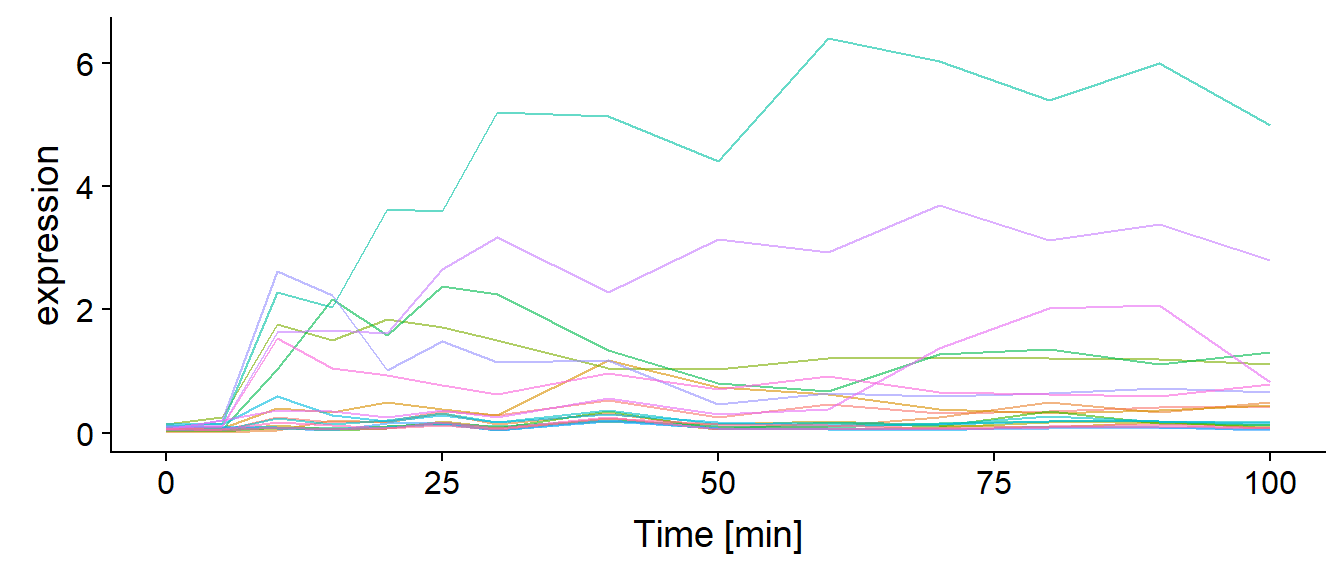# Basics of The Model

In all of the following, $$x_i$$ is the true (unknown) expression of a target gene, $$y_j$$ the true protein concentration of a regulator and $$\rho_i$$ the regulatory input for the target gene. Both $$x_i$$, $$y_j$$ and $$\rho_i$$ are functions of time while all other parameters are constants. The regulatory input is a linear combination of protein concentrations of the regulators:

$\rho_i = \sum_j{w_{i,j}y_j} + b_i$ Then the expression of $$x_i$$ is driven by the following ordinary differential equation (ODE): \begin{align} \frac{\mathrm{d}x_i}{\mathrm{d}t} &= s_i F(\rho_i) - d_i x_i \\ F(\rho_i) &= \frac{1}{1 + e^{-\rho_i}} \end{align}

where $$F$$ is the regulatory response function, in our case the logistic sigmoid.

Our goal is to use Stan to infer the parameters of this model ($$s_i,w_{i,j},b_i, d_i$$), given the observed expression of the target genes and regulators over time. The model assumes constant degradation ($$d_i$$) over time which is unrealistic, but necessary as most datasets do not contain enough information to identify varying degradation.

There are two forms of expression data available with widely different noise models: microarray and RNA-seq. The former produces positive continuous measurements with approximately normal error while the latter produces count data with negative-binomial distribution. The datasets we are interested in come from microarray experiments so we will focus on those, but using RNA-seq means just changing the observation model. For microarrays, the observation model can be treated as a truncated normal:

$\tilde{x_i}(t) \sim N(x_i(t), \sigma_{abs} + x_i(t)\sigma_{rel}) \mathop{|} \tilde{x_i}(t) > 0$

The two error terms represent the fixed absolute error ($$\sigma_{abs}$$) which is the result of technical noise in the microarray platform which is constant (Klebanov and Yakovlev 2007) and the relative error ($$\sigma_{rel}$$) wich represents the uncaptured biological variation which tend to be proportional to the expresssion of the genes.

For the regulator(s) there are two possible regimes: a) the expression of the regulators is observed and the protein concentration is assumed to be identical to the true expression or b) the $$y$$ is estimated only through its influence on $$x$$. In case a), the exact same error model applies to regulators.

In the following, we treat both $$\sigma_{abs}$$ and $$\sigma_{rel}$$ as given. The model can treat the error terms as parameters, but with the dataset we work with, the resulting $$\sigma_{rel}$$ values tended to be unrealistically high and most fits unexpectedly poor as a consequence. We are yet to investigate why this is the case.

Based on our previous experience with similar models, we expect $$\sigma_{abs} = 0.1$$ and the biological variation to be around 20% of the expression, suggesting $$\sigma_{rel} = 0.1$$.

# Modelling Regulator Expression

To solve the regulation ODE numerically, we need to have $$y_j$$ available at much finer intervals than the available measurements (1-2 minute intervals have proven sufficient). Initially, we considered Gaussian Processes (as Titsias et al. 2012)), but those introduced computational issues and we switched to B-splines which have less theoretical appeal, but introduce fewer parameters and were easier to fit.

We however need to ensure that $$y_j$$ are positive. After some early setbacks with the log1pexp transform ($$x^+ = ln(1+e^x)$$), we settled to simply subtract the minimum of the spline from all points1, i.e. given the spline basis $$B$$ we get:

\begin{aligned} \bar{y_j} &= B\alpha_j \\ y_j &= c_{scale}(\bar{y_j} - \min{\bar{y_j}}) + \beta_j \\ \alpha_j &\sim N(0,1) \\ \beta_j &\sim HalfNormal(0, \sigma_\beta) \end{aligned}

Where $$\alpha_j$$ is a column vector of spline coefficients and $$\beta_j$$ serves as intercept, all are treated as parameters. The scaling constant $$c_{scale}$$ and $$\sigma_\beta$$ reflect the range of the data and are given by the user. When the regulator proteins are estimated from the effect on targets only (no expression measurements for regulator), the spline intercept $$\beta_j$$ is ignored, as it cannot be separated from $$b_i$$.

# Solving the ODE

It is a bit tricky to use Stan’s built-in ODE solver with a spline as one of the parameters driving ODE evolution as it needs the value of $$y_i$$ at arbitrary time points. The most straightforward way - linearly interpolating between precomputed discrete values of $$y_i$$ requires some hacks and breaks the solver. In principle, $$\alpha_i$$ could be given to the ODE solver and the spline basis computed for each timepoint the solver needs, but this seemed cumbersome. Instead we decided to follow the approach of (Titsias et al. 2012). First we can observe that the regulation ODE is linear in $$x_i$$ and can be solved for $$t \geq 0$$:

$x_i(t) = \eta_i e^{-d_i t} + s_i \int_0^t F(\rho_i(u)) e^{-d_i(t-u)} \mathrm{d}u$ Where $$\eta_i$$ is the concentration of the target at $$t=0$$. We then solve the resulting integral numerically via trapezoid rule2. Assuming unit timestep, $$x_i$$ can be computed in a single for loop:

\begin{aligned} \chi_i(0) &= -\frac{1}{2} F(\rho_i(0)) \\ \chi_i(t + 1) &= (\chi_i(t) + F(\rho_i(t)))d_i \\ x_i(t) &= \eta_i e^{-d_i t} + s_i(\chi_i(t) + \frac{1}{2}F(\rho_i(t))) \end{aligned}

# Identifiability

The model as given above is biologically relevant, but has multiple identifiability issues, all of which can be demonstrated with just a single regulator and a single target, so we will drop parameter indicies for the rest of this section. The first non-identifiability arises with $$w$$ and $$b$$ if all values of $$\rho$$ fall on the tail of the sigmoid, which is approximately linear. In this case, the walue of $$F(\rho)$$ becomes insensitive to linear transformations of $$w$$ and $$b$$:

time <- seq(0,2,length.out = 100)
regulator <- sin(time * 4) + 1

params1 <- c(degradation = 0.5, bias = -3, sensitivity = 1, weight = 5, basal_transcription = 0, protein = approxfun(time, regulator, rule=2));
target1 <-  ode( y = c(x = 0), times = time, func = target_ODE, parms = params1, method = "ode45")[,"x"];

params2 <- c(degradation = 0.5, bias = -6, sensitivity = 1, weight = 10, basal_transcription = 0, protein = approxfun(time, regulator, rule=2));
target2 <-  ode( y = c(x = 0), times = time, func = target_ODE, parms = params2, method = "ode45")[,"x"];

params3 <- c(degradation = 0.5, bias = -30, sensitivity = 1, weight = 50, basal_transcription = 0, protein = approxfun(time, regulator, rule=2));
target3 <-  ode( y = c(x = 0), times = time, func = target_ODE, parms = params3, method = "ode45")[,"x"];

params_to_legend <- function(params) {
paste0("w = ", params["weight"], ", b = ", params["bias"])
}

data.frame(time = time, regulator = regulator, target1 = target1, target2 = target2, target3 = target3) %>%
gather("profile","expression", -time) %>%
ggplot(aes(x = time, y = expression, color = profile)) +
geom_line() +
scale_color_hue(labels = c("regulator", params_to_legend(params1), params_to_legend(params2), params_to_legend(params3))) + ggtitle("Non-identifiability due to w and b","All targets have s = 1, d = 0.5")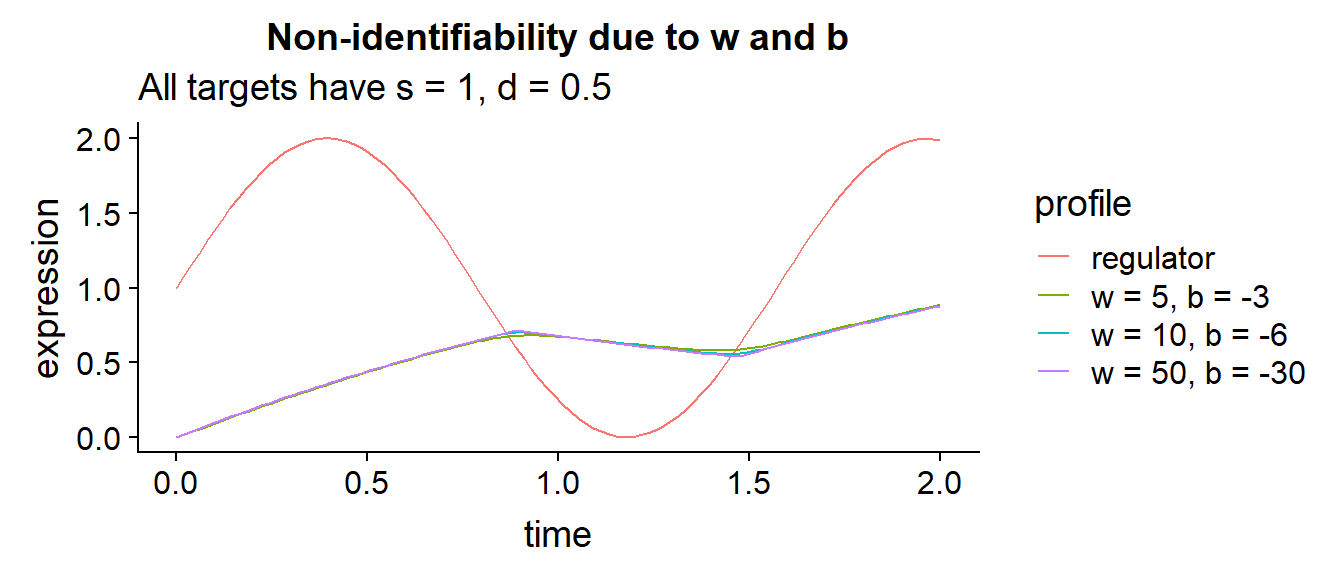Transformations of $$s$$ and $$d$$ together may also have very minor effect on the resulting expression. While the resulting expression profiles are more different than in the previous case, those differences are still very small compared to the noise observed in real data. In the following figures, the red dots represent measurements that have approximately equal likelihood for each of the shown true target profiles.

time <- seq(0,2,length.out = 100)
regulator <- sin(time * 4) + 1

params1 <- c(degradation = 5, bias = -1, sensitivity = 10, weight = 1, basal_transcription = 0, protein = approxfun(time, regulator, rule=2));
target1 <-  ode( y = c(x = 1), times = time, func = target_ODE, parms = params1, method = "ode45")[,"x"];

params2 <- c(degradation = 10, bias = -1, sensitivity = 19, weight = 1, basal_transcription = 0, protein = approxfun(time, regulator, rule=2));
target2 <-  ode( y = c(x = 1), times = time, func = target_ODE, parms = params2, method = "ode45")[,"x"];

params3 <- c(degradation = 100, bias = -1, sensitivity = 180, weight = 1, basal_transcription = 0, protein = approxfun(time, regulator, rule=2));
target3 <-  ode( y = c(x = 1), times = time, func = target_ODE, parms = params3, method = "ode45")[,"x"];

params_to_legend <- function(params) {
paste0("s = ", params["sensitivity"], ", d = ", params["degradation"])
}

measured_data = data.frame(profile = "measured", time = seq(0,2,length.out = 9),
expression = c(1,1.7,1.4,0.85, 0.83,0.35,0.7,1.4,1.5))

data.frame(time = time, regulator = regulator, target1 = target1, target2 = target2, target3 = target3) %>%
gather("profile","expression", -time) %>%
ggplot(aes(x = time, y = expression, color = profile)) + geom_line() +
geom_point(data = measured_data, color = "#ba1b1d", size = 3) +
scale_color_hue(labels = c("regulator",params_to_legend(params1), params_to_legend(params2), params_to_legend(params3))) + ggtitle("Non identifiability due to s and d","All targets have w = 1, b = -1")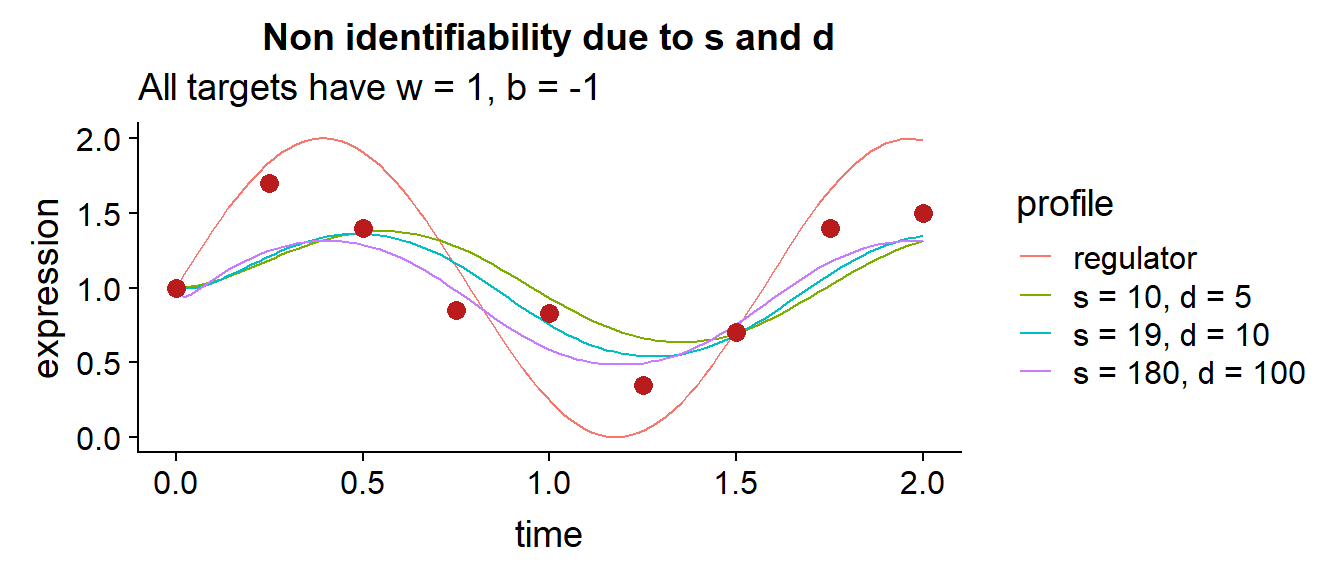There is also a non-identifiability when $$w = 0$$ as $$s$$ and $$b$$ become redundant. Even worse, some of the solutions with $$w = 0$$ might not be distinguishable form solutions with $$|w| \gg 0$$. This might also induce non-identifiability in the initial condition $$\eta$$ as it is much less constrained by data. Once again the expression profiles are not identical, but are close enough that given the amount of noise, non-negligible posterior mass can be found for all of them:

time <- seq(0,2,length.out = 100)
regulator <- sin(time * 4) + 1

params1 <- c(degradation = 3, bias = -1, sensitivity = 3, weight = 5, basal_transcription = 0, protein = approxfun(time, regulator, rule=2));
target1 <-  ode( y = c(x = 1.5), times = time, func = target_ODE, parms = params1, method = "ode45")[,"x"];

params2 <- c(degradation = 3.4, bias = 0, sensitivity = 6, weight = 0, basal_transcription = 0, protein = approxfun(time, regulator, rule=2));
target2 <-  ode( y = c(x = 1.6), times = time, func = target_ODE, parms = params2, method = "ode45")[,"x"];

params3 <- c(degradation = 10, bias = 0, sensitivity = 16, weight = 0, basal_transcription = 0, protein = approxfun(time, regulator, rule=2));
target3 <-  ode( y = c(x = 2.5), times = time, func = target_ODE, parms = params3, method = "ode45")[,"x"];

params_to_legend <- function(params) {
paste0("s = ", params["sensitivity"],", w = ", params["weight"], ", b = ", params["bias"], ", d = ", params["degradation"])
}

measured_data = data.frame(profile = "measured", time = seq(0,2,length.out = 9),
expression = c(1.8,0.9,1,0.66, 1.11,0.6,0.7,0.96,1.03))

data.frame(time = time, regulator = regulator, target1 = target1, target2 = target2, target3 = target3) %>%
gather("profile","expression", -time) %>%
ggplot(aes(x = time, y = expression, color = profile)) + geom_line() +
geom_point(data = measured_data, color = "#ba1b1d", size = 3) +
scale_color_hue(labels = c("regulator",params_to_legend(params1), params_to_legend(params2), params_to_legend(params3))) + ggtitle("Non identifiability between w=0 and w > 0")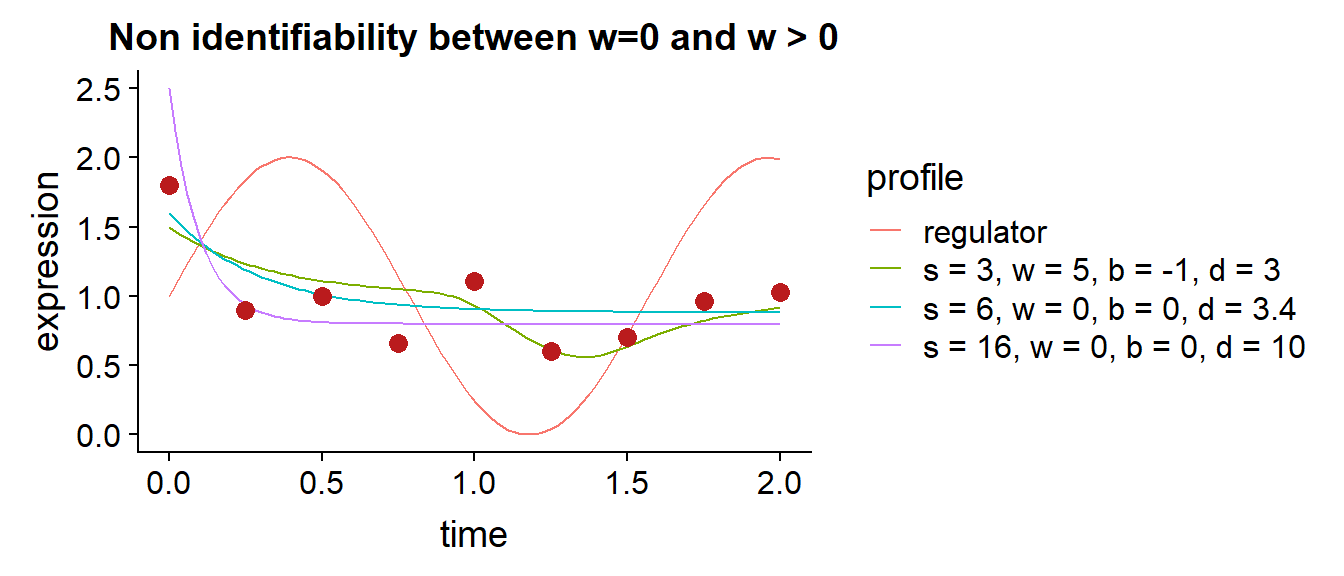Last but not least, it is also possible to get similar solutions with different sign of $$w$$:

time <- seq(0,2,length.out = 100)
regulator <- c(0.3296698,0.6667181,1.0083617,1.3518170,1.6943005,2.0330289,2.3652186,2.6880862,2.9988482,3.2947213,3.5729219 ,3.8306665,4.0651718,4.2736542,4.4533304,4.6014168,4.7154822,4.7961600,4.8456619,4.8662125,4.8600365,4.8293585 ,4.7764032,4.7033952,4.6125592,4.5061198,4.3863016,4.2553294,4.1154277,3.9688212,3.8177346,3.6643924,3.5110195 ,3.3598403,3.2130795,3.0725728,2.9385992,2.8110488,2.6898117,2.5747779,2.4658374,2.3628803,2.2657967,2.1744767 ,2.0888102,2.0086873,1.9339981,1.8646326,1.8004810,1.7414331,1.6873792,1.6382092,1.5938132,1.5540812,1.5189034 ,1.4881697,1.4617702,1.4395950,1.4215341,1.4074776,1.3973155,1.3909378,1.3882347,1.3890962,1.3934123,1.4010732 ,1.4119687,1.4259843,1.4428964,1.4623727,1.4840759,1.5076691,1.5328149,1.5591763,1.5864163,1.6141975,1.6421830 ,1.6700355,1.6974179,1.7239932,1.7494242,1.7733737,1.7955046,1.8154798,1.8329622,1.8476146,1.8590998,1.8670809 ,1.8712205,1.8711817,1.8666272,1.8572200,1.8426229,1.8224987,1.7965104,1.7643208,1.7255927,1.6799891,1.6271729 ,1.5668068) * 0.5

params1 <- c(degradation = 10, bias = -8, sensitivity = 15, weight = 10, basal_transcription = 0, protein = approxfun(time, regulator, rule=2));
target1 <-  ode( y = c(x = 0), times = time, func = target_ODE, parms = params1, method = "ode45")[,"x"];

params2 <- c(degradation = 1, bias = 3, sensitivity = 100, weight = -10, basal_transcription = 0, protein = approxfun(time, regulator, rule=2));
target2 <-  ode( y = c(x = 0), times = time, func = target_ODE, parms = params2, method = "ode45")[,"x"];

params_to_legend <- function(params) {
paste0("s = ", params["sensitivity"],", w = ", params["weight"], ", b = ", params["bias"], ", d = ", params["degradation"])
}

measured_data = data.frame(profile = "measured", time = seq(0,2,length.out = 9),
expression = c(0.05,1.52,1.44,0.83, 0.96,1.01,0.72,1.07,0.65))

data.frame(time = time, regulator = regulator, target1 = target1, target2 = target2) %>%
gather("profile","expression", -time) %>%
ggplot(aes(x = time, y = expression, color = profile)) + geom_line() +
geom_point(data = measured_data, color = "#ba1b1d", size = 3) +
guides(color=FALSE) + ggtitle("Non identifiability between w < 0 and w > 0")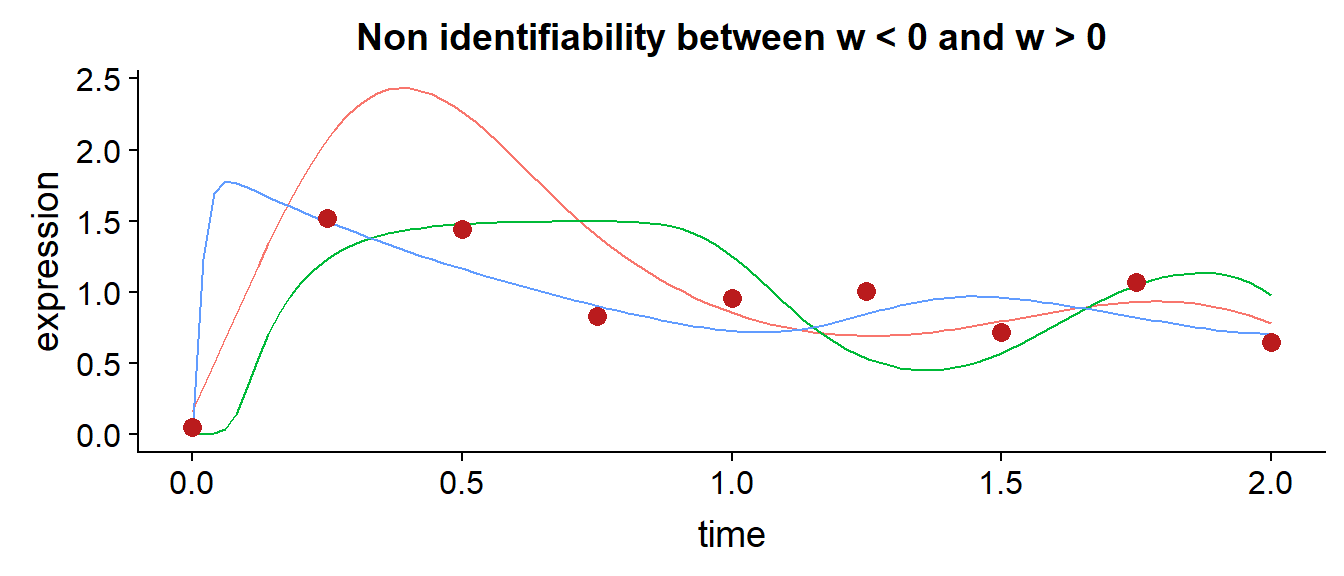There are probably many more non-identified scenarios, especially when multiple targets are involved, but the above are of the type we have actually encountered with real data.

## Reparametrization

First we realized that when there is a good fit with $$w < 0$$ and a similarly good one with $$w > 0$$, there is nothing we can do to make the model identifiable, so we decided to make the user specify the signs of regulatory interactions $$I_{i,j} = sgn(w_{i,j})$$ as data. This is not a big hindrance as this type of regulation model is mostly employed to test direct regulations by sigma factors which are expected to always have $$w > 0$$. If the sign of $$w$$ is important and there is only one regulator, it is possible to fit each target separately with different values of $$I_{1,1}$$. The only truly problematic case is when fitting a model with multiple regulators and unknown $$I$$, but since two regulators already often overfit on most practical datasets this is of little practical concern. Additionally, user may set some $$I_{i,j} = 0$$ to indicate known absence of regulation.

Some of the non-identifiabilities arise because certain simple aspects of the resulting expression (e. g. magnitude) are influenced by multiple parameters. To get rid of those complex dependencies, we introduced several reparametrizations. The only parameter we kept directly is the degradation parameter $$d_i$$, which also has the nice property that it does not depend on the scale of the expression data (only on the length of the timestep).

Instead of $$s_i,b_i$$ and $$w_{i,j}$$, we introduce $$\mu^{\rho}_i$$, the mean regulatory input, $$\sigma^{\rho}_i$$, the sd of the regulatory input, $$\gamma_i$$ a simplex of relative regulator weights and $$a_i$$ the asymptotic normalized state. All of those parameters are also decoupled from the actual values of the expression data. Formally:

\begin{align} \mu^{\rho}_i &= E(\rho_i) \\ \sigma^{\rho}_i &= \mathrm{sd}(\rho_i) \\ a_i &= \frac{s_i E(F(\rho_i))}{d_i \max{\tilde{x_i}}} \\ \end{align}

Where $$E$$ and $$\mathrm{sd}$$ correspond to the sample mean and standard deviation. Solving for the original parameters we get:

\begin{align} w_{i,j} &= I_{i,j} \gamma_{i,j} \frac{\sigma^{\rho}_i}{\mathrm{sd}(y_j)} \\ b_i &= \mu^{\rho}_i - \sum_j w_{i,j} E(y_j) \\ s_i &= \frac{a_i d_i \max\tilde{x_i} }{ E(F(\rho_i)) } \\ \sum_j \gamma_{i,j} &= 1 , & \gamma_{i,j} > 0 \\ \end{align} The formula for $$w_{i,j}$$ assumes independence among the regulators which does not hold, but handling the covariance structure correctly would be difficult and could lead to multiple solutions. The interpretion of $$a_i$$ is that if the expression stays at its mean level, e.g. $$F(\rho_i) \simeq E(F(\rho_i))$$ and $$t \rightarrow \infty$$ then $$x_i(t) \rightarrow a_i \max\tilde{x_i}$$. In other words $$a_i$$ is related to the hypothetical steady state long time in the future. Nevertheless the cells do not reach steady state for this datasets (and indeed for most datasets). Also note that using $$\max\tilde{x_i}$$ to scale $$s_i$$ means the model is not completely generative, as we cannot generate $$\tilde{x_i}$$ before we generate $$s_i$$, but this has shown to not be a problem in practice.

The reparametrization not only helped identify the posterior but it is also much easier to specify priors for those parameters! We ended up with the following priors: \begin{align} \mu^{\rho}_i &\sim N(0,\tau_{\rho,\mu}) &\\ \sigma^{\rho}_i &\sim N(0,\tau_{\rho,\sigma}) &| \sigma^{\rho}_i > 0 \\ a_i &\sim N(1,\tau_{a}) &| a_i > 0 \\ log(d_i) &\sim N(\nu_d,\tau_d) & \end{align} Where all the hyperparameters are given by user, but sensible defaults can be given as the hyperparameters are decoupled from the scale of the data. Since the sigmoid $$F$$ is mostly saturated outside $$[-5,5]$$, we use $$\tau_{\rho,\mu} = \tau_{\rho,\sigma} = 5$$. Further we expect $$a_i$$ should lie mostly in $$[0,2]$$ (e.g. if given more time with similar regulatory input the gene will unlikely raise to more than twice the maximum observed), giving $$\tau_a = \frac{1}{2}$$ and finally we expect degradation to be non-negligible but less than 1 (e.g. all mRNA degrading in a single unit of time), giving $$\nu_d = -2$$ and $$\tau_d = 1$$.

## The constant synthesis model

To get rid of the non-identifiabilities connected with $$w = 0$$, we first try to fit a simpler constant synthesis model to each target separately. This model is given by:

$\frac{\mathrm{d}x_i}{\mathrm{d}t} = s'_i - d'_i x_i$ As in the general model, the constant synthesis model is not always well identified with this representation and we thus reparametrize with $$d'_i$$ and $$a'_i$$ as the asymptotic normalized state.

$s'_i = a'_i d'_i \max\tilde{x_i}$ This also lets us use the same priors for $$a'_i$$ and $$d'_i$$ as for $$a_i$$ and $$d_i$$ respectively.

If the target is “reasonably well” fit with the constant synthesis model, it is not considered for the full model, because it could then be fit by any regulator, simply be setting $$w = 0$$. The quality of the model fit is assessed with looic, see discussion below for further details. Note that the constant synthesis model also fits all lowly expressed genes. To our knowledge, filtering with a such a simpler model was first proposed in our recent work (Modrák and Vohradský 2018).

# Workflow

The assumed workflow when using the model to infer novel regulations proceeds as follows:

1. Gather putative and known targets of the regulators of interest
2. Fit the constant synthesis model to all known & putative targets
3. Assess model fit and discard targets that are fit well
4. Optional: Use the known targets to constrain the true expression of the regulators
5. Fit the main model to each putative target separately
6. Compare the fit with the main model to the fit of the constant synthesis model

In the following example, we will use 5 known and 4 putative targets of the sigA regulator. We use cubic spline with 6 degrees of freedom, $$c_{scale} = 5$$ as the basis for the main model.

#Globally used params for the algorithm
measurement_times = gse6865_raw_time + 1
smooth_time  <- 1:101#seq(0,100, by = 1)
expression_data <- gse6865_raw

spline_df <- 6
spline_basis <- bs(smooth_time, degree = 3, df = spline_df)
default_spline_params <- spline_params(
spline_basis = spline_basis,
scale = 5
)

default_params_prior <-  params_prior(
initial_condition_prior_sigma = 2,
asymptotic_normalized_state_prior_sigma = 2,
mean_regulatory_input_prior_sigma = 5,
sd_regulatory_input_prior_sigma =5,
intercept_prior_sigma = 2
)

default_measurement_sigma = measurement_sigma_given(0.1,0.1)
putative_targets <- c("kinE","purT", "yhdL", "codV")

#The known targets are those predicted and biologically validated in our previous work with the dataset (https://doi.org/10.1016/j.bbagrm.2017.06.003)
known_targets <- c("acpA","fbaA","rpmGA","ykpA","yyaF")

plot_profiles <- function(expression_data, targets) {
expression_data[targets,,drop = FALSE] %>% as.data.frame() %>%
rownames_to_column("gene") %>%
gather("time","expression",-gene) %>%
mutate(time = as.integer(gsub("min","",time, fixed = TRUE))) %>%
ggplot(aes(x = time, y = expression, color = gene, linetype = gene)) + geom_line()
}

plot_profiles(expression_data, "sigA") + ggtitle("Regulator")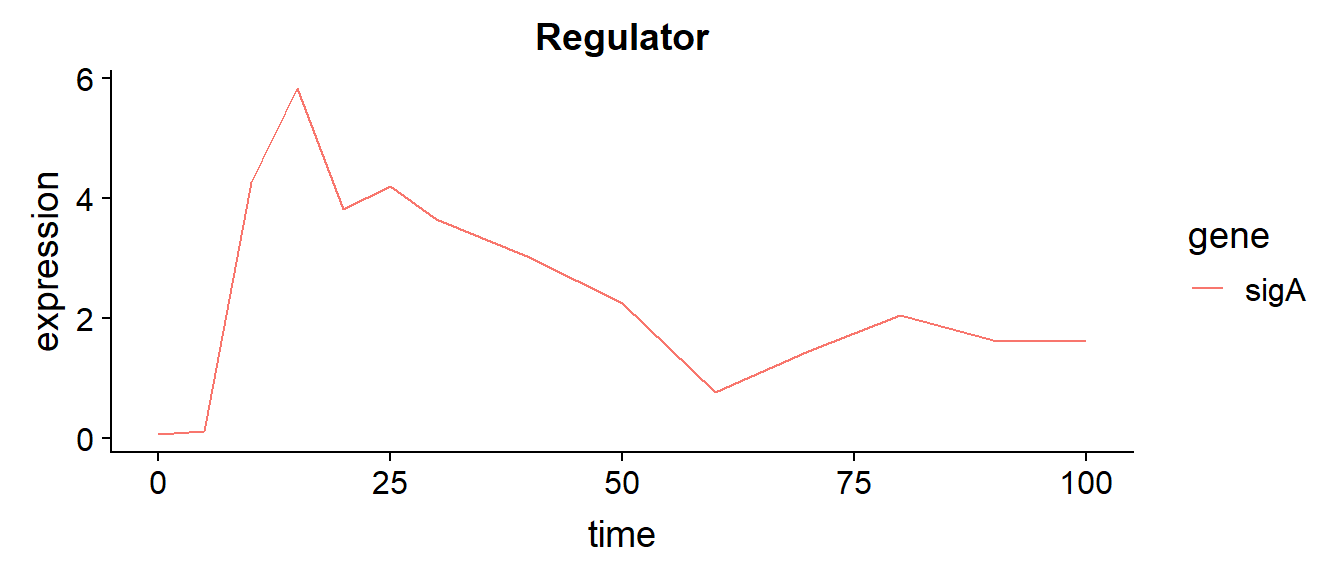plot_profiles(expression_data, known_targets) + ggtitle("Known targets")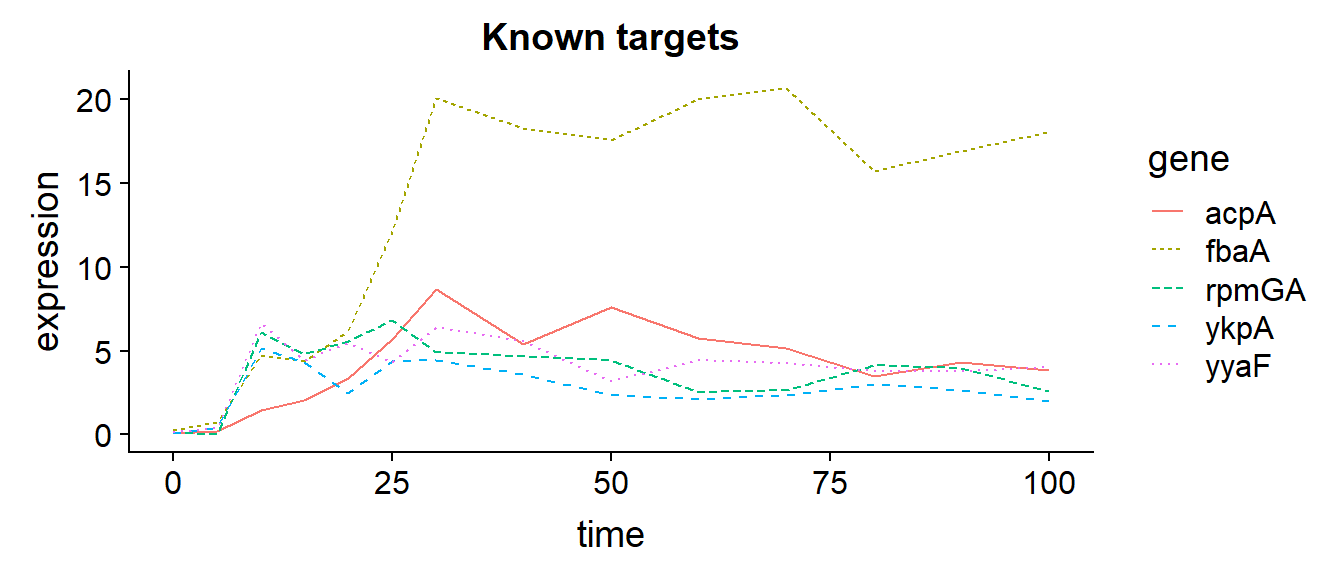plot_profiles(expression_data, putative_targets) + ggtitle("Putative targets")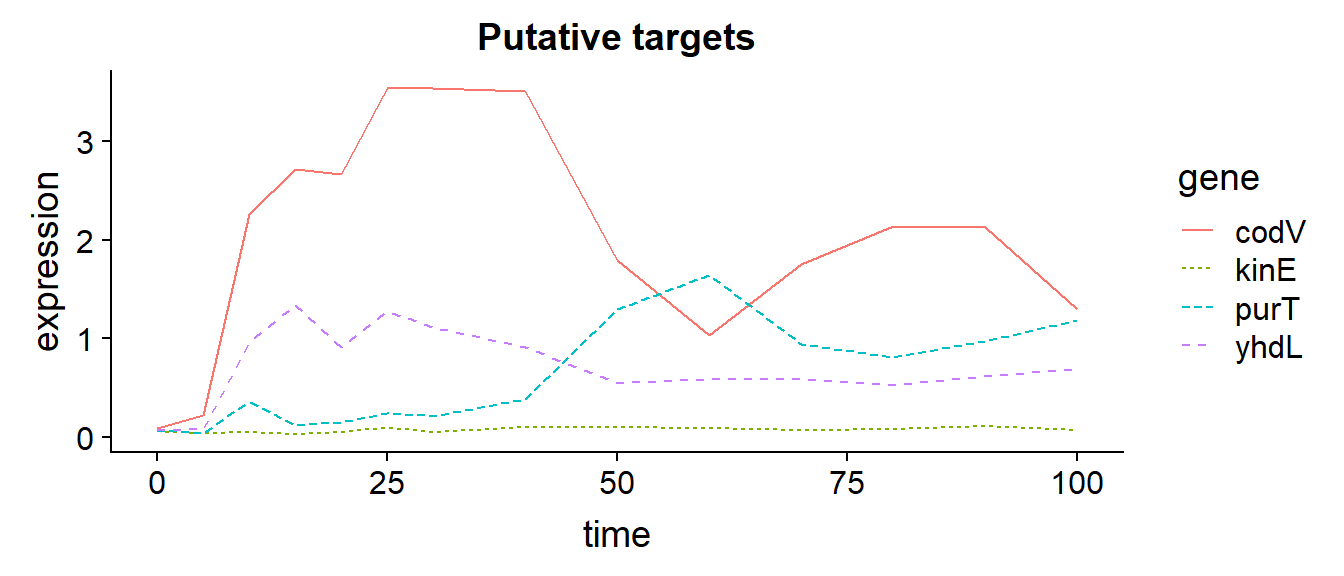## Fit the constant synthesis model

Below are posterior samples from fitting the constant synthesis model to the putative targets.

In addition to samples from posterior true expression we also show simulated replicates of the measured values, to get a better sense of the level of noise expected by the model.

csynth_model <- stan_model(file = here('stan','constant_synthesis.stan'))
targets <- c(putative_targets)
data_csynth <- list()
fits_csynth <- list()
loo_csynth <- list()
for(t in 1:length(targets)) {
data_csynth[[t]] <- list(
num_measurements = length(measurement_times),
measurement_times = measurement_times,
expression = expression_data[targets[t],],
measurement_sigma_absolute = default_measurement_sigma$sigma_absolute_data, measurement_sigma_relative = default_measurement_sigma$sigma_relative_data,
initial_condition_prior_sigma = default_params_prior$initial_condition_prior_sigma, asymptotic_normalized_state_prior_sigma = default_params_prior$asymptotic_normalized_state_prior_sigma,
degradation_prior_mean = default_params_prior$degradation_prior_mean, degradation_prior_sigma = default_params_prior$degradation_prior_sigma
)
fits_csynth[[t]] <- sampling(csynth_model, data_csynth[[t]])
loo_csynth[[t]] <- get_loo_csynth(fits_csynth[[t]])
}
plots <- list()
for(t in 1:length(targets)) {
fit <- fits_csynth[[t]]
plot1 <- fitted_csynth_plot(fit, data_csynth[[t]], name = targets[t])
plot2 <- fitted_csynth_observed_plot(fit, data_csynth[[t]], name = targets[t])
plot_grid(plot1, plot2, ncol = 2) %>% print()
}`